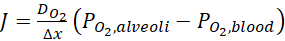1. Science
2. Biology
3. we need to calculate the diffusion rate for oxygen from...

# Question: we need to calculate the diffusion rate for oxygen from...

###### Question details

We need to calculate the diffusion rate for oxygen from the lungs to the bloodstream, as a function of "external" variables, such as air pressure and "internal" variables, such as anatomical and physiological parameters. We will also determine how the oxygen transport changes when these parameters are altered (e.g., under various diseases of extreme conditions). Here, "arterial" means the oxygenated blood in the systemic circulation.

Assume the following (unless noted otherwise in the problem)

• The cardiac output (blood volumetric flow rate) is 5L/min
• Breathing rate is 12 breaths per minute
• Oxygen makes up 21% by volume of the inhaled air (which can be considered an ideal gas)
• The inhaled air is at atmospheric pressure (760 mmHg)
• Air in the alveoli has a pressure which is 62.3% of the inhaled air
• Oxygen diffuses out of the alveoli into the blood according to Fick’s Law, which we will write as J=DO2∆xPO2, alveoli-PO2, blood, where DO2 = 1.3e-7 mL/(min*mmHg*m) and includes the Henry’s law constants relating pressure and concentration.
• The surface area of the alveoli available for gas exchange is 75 m2
• The thickness of the membrane between alveolar air and the capillary is 0.5 um
• The partial pressure of the O2 in the de-oxygenated blood in the lung is initially 40 mmHg (before O2 transport)
• The partial pressure of the O2 in the blood after O2 transport is the same as the partial pressure of O2 in the lungs

For the conditions, given below, calculated the following:

1. For the normal individual, what is the rate of oxygen transported from the lungs to the blood, in mL/min?
2. You are a mountain climber, scaling Mount Everest. The air pressure at the summit (8848m, or 28,750 ft.) is 253 mmHg. What are the alveolar and arterial blood PO2 values?
3. Venous blood PO2 drops to about 20 mmHg at this altitude. What is the rate of oxygen transported from the lungs to the blood, in mL/min?
4. An often serums consequence of high-altitude mountaineering is pulmonary edema, or excessive fluid volume in the interstitial space between the alveoli and capillary. Pulmonary edema can also occur at normal altitude (i.e., sea level), if pulmonary blood pressure rises for some reason, such as left ventricular failure. The effect of pulmonary edema is to increase the diffusion distance. Suppose that the distance between the alveoli and the capillary increases to 1um (but all other values remain normal). What is the rate of oxygen transported from the lungs to the blood, in mL/min?
5. In emphysema, enzymes destroy the elastic fibers of the lung and kill cells, breaking down the walls of the alveoli. This results in a lung with fewer and larger alveoli; consequently, the surface available for gas exchange decreases to about 70% of normal. What is the rate of oxygen transported from the lungs to the blood, in mL/min?
###### Solution by an expert tutor